# Mixed Numbers, Simplification of Fractions and Improper Fractions

Before you tackle the examples below, have a read of our Fractions - Proportions of Numbers page. It’s packed with helpful advice on how to best teach your child about fractions.

## Simplification of Fractions

The use of fractions puts a lot of children off maths but they are not as intimidating as you may think. Fractions at primary school level are not likely to get very complicated and 11+ papers will not be much more challenging.

A key issue to remember when looking at a fraction is that it is a number, but is also a sum. That line down the middle is the equivalent of a division sign.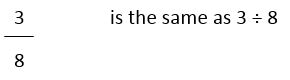This means that there are a lot of fractions that are identical. For example, if you divide 1 by 2, you get the same answer as if you divide 4 by 8.

That means that 1 ÷ 2 = 4 ÷ 8, or, in fractions,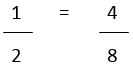Often a complicated-looking fraction can be shown in a far simpler format. We can deal with a half - we know what that looks like - whereas someone talking about seven fourteenths of a figure would have some of us worrying. There is an easy way to simplify complicated fractions and make them a lot more manageable.

Firstly, look at the two numbers on top and bottom of the fraction. They are called the numerator and denominator respectively.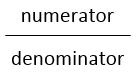If the numerator is 1, you can't simplify the fraction. It is already in its simplest form. However, if you can divide both the numerator and denominator by the same number, you can simplify the fraction.

Let's take, as an example, four twentieths. Which number goes into both top and bottom?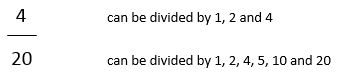Ignore the fact that they can be divided by 1 - every number can - find a number that both numbers can be divided by. The larger, the better. If you find the largest one straight away, you'll save yourself repeating the whole process.

In our example, the highest number that both numerator and denominator can be divided by is 4. We'll now divide the numerator and denominator by 4...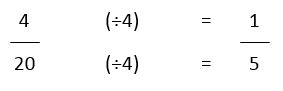This shows that the fractions are the same as each other. Four twentieths is the same as one fifth.

So how do you know if it is in its simplest form? The best way to tell is to look for a prime number - if one of the numbers is a prime (nothing can divide into it other than one and itself) then the fraction is likely to be in its simplest form or you can only use that prime number to divide by to get a simpler form. For example, five is a prime number. Only one and five fit into it.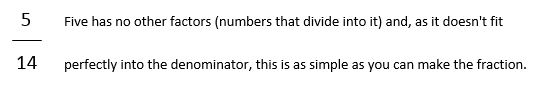However...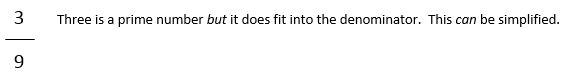A good tip is to look for even numbers. If both numerator and denominator are even, at least you can divide by two. Sometimes it's easier to do the process repeatedly and get the answer right rather than try to find the highest number that fits into both, get a calculation wrong, and mess up the question.

## Mixed Numbers and Improper Fractions

Fractions which are presented in a recognisable form with a small number over a bigger number are proper fractions. They are equal to less than a whole one. However, there are times when it is necessary to use either an improper fraction, where the number on top is larger than the one below, or a mixed number, which includes a whole number alongside a proper fraction. Improper fractions sometimes get called top-heavy fractions.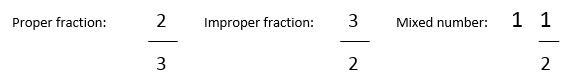The proper fraction is two thirds. The other two are both one and a half - but they are presented in different ways. If you divide 3 by 2, which is what the improper fraction above means, then you get one and a half. This is a mathematically neat way of showing things but of course we are more familiar with seeing the other format, with the whole number followed by the fraction.

There is a straightforward way to convert an improper fraction into a mixed number. Look at how many times the bottom number (denominator) fits into the top number (numerator). That's the number of whole ones in the answer. The remainder, if there is one, will go over the denominator as a fraction.

For instance...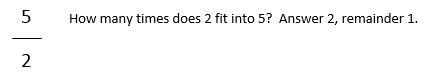The answer will therefore be 2, as the whole number, and 1 over 2 (remainder over the denominator) as the fraction. That's two and a half.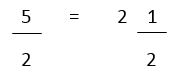[Notice that the denominator remains the same; however, the fraction part of the mixed number may need to be simplified to show it in its most recognisable form.]

Now, the same works in reverse. If you need to change a mixed number into an improper fraction then multiply the denominator by the whole number and add the numerator. Put this figure over the denominator.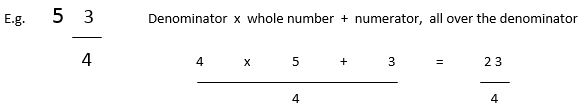We use cookies to make your experience of our website better.

To comply with the new e-Privacy directive, we need to ask for your consent -